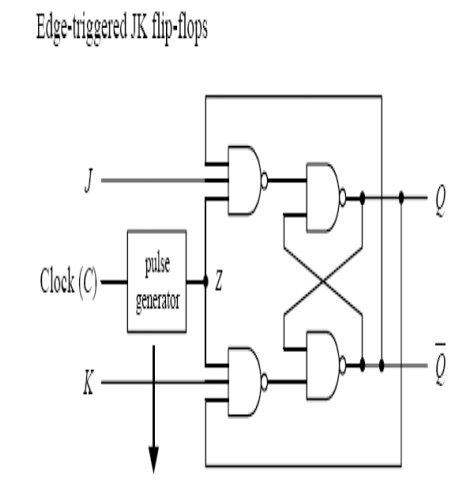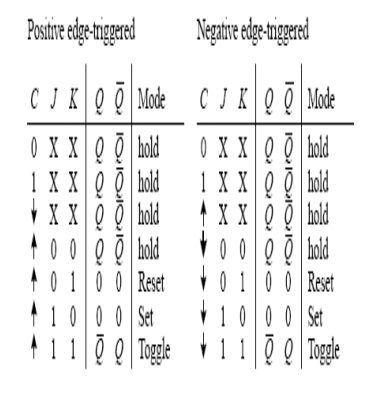Home | | Digital Electronics | JK Flip-Flops

# JK Flip-Flops

Proposed to get rid of the forbidden I/P problem of R-S

JK FLIP-FLOPS:

Proposed to get rid of the forbidden I/P problem of R-S

I) J=1, K=0:

(a) Let Q=1, → R=0, S=0

→ Hold state of R-S → Q=1, Q =1 Q = 0

Q = 0 Q = 0

ii)          J=0, K=1  Q=0, using a similar analysis

iii)        J=K=0 ≡ Hold state

A JK flip-flop resembles an SR flip-flop, where J acts like S and K acts like R. Likewise, it has a set mode (J = 1, K = 0), a reset mode ( J = 0, K = 1), and a hold mode ( J = 0, K = 0). However, unlike the SR flip-flop, which has an indeterminate mode when S = 1, R = 1, the JK flip-flop has a toggle mode when J = 1, K = 1. Toggle means that the Q and Q_ outputs switch to their opposite states at each active clock edge. To make a JK flip-flop, modify the SR flip flop’s internal logic circuit to include two cross-coupled feedback lines between the output and input. This modification, however, means that the JK flip-flop cannot be level-triggered; it can only be edge-triggered or pulse-triggered. Fig shows how you can create edge-triggered flip-flops based on the cross-NAND SR edge triggered flip-flop.Study Material, Lecturing Notes, Assignment, Reference, Wiki description explanation, brief detail
Digital Electronics : Sequential Circuits : JK Flip-Flops |